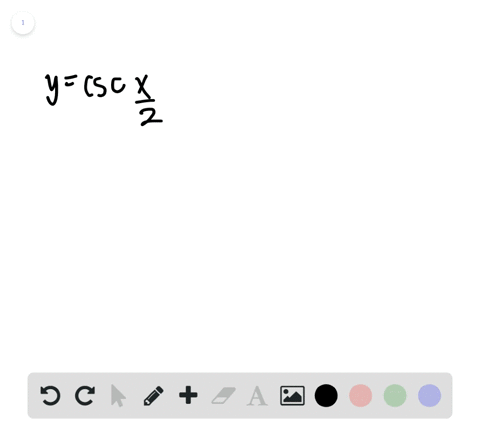🎉 The Study-to-Win Winning Ticket number has been announced! Go to your Tickets dashboard to see if you won! 🎉View Winning Ticket### Sketching the Graph of a Trigonometric Function I…

00:29University of Southern California
Problem 58

# Sketching the Graph of a Trigonometric Function In Exercises $55-66,$ sketch the graph of the function.$$y=2 \tan x$$

## Discussion

You must be signed in to discuss.

## Video Transcript

problem. Number 58 asks us to create a sketch of the following function. Why equals to tension of X? Now, before we begin, I want to point out that why equals Tangent of X is also equal to sign of X over coastline of X. And this breaking the formula done into this is really important because it allows us to understand a really, uh, characteristic aspect of tangents. Graff And that is that tangent has Assam a totes in its graph and ask him a toaster points where our function does not have a defined value. And so when we look at our this expression for tangent sine over co sign, we see that because there is co sign of the denominator whenever it's equal to pi halfs, it's going to be undefined because cosign a pi house zero. So I'm gonna go through here and break up our number line into increments of pi house and because we know already know that at these points our function is going to be undefined. I'm gonna go ahead and draw some dotted lines just so we have a better visual of these ass mottos. So this is the first crucial part of drawing this function. They're with me here while I get these lines in. All right, That should be good now, because there's nothing inside of our triggered a metric function y equals tangent of X. We know that the period is just going to be the standard period of, um hi. Because the period of Tanja is equal too high over the absolute value of being and in this instance be is one. So our period is pie, huh? I just realized I made an error. Excuse that, please. So, yes, at increments of pie and two point, there will not be as mottos sound. It's a good thing to catch. And now if we look at this other term, our this other aspect of our equation this too. We see that it's multiplied toward Tanja necks. And when we have graft signing coastline, we see that that changes the amplitude. But in the context of tangent, it doesn't necessarily change the amplitude because tangent extends both from negative infinity operate a positive infinity so that to that constant of the front is pretty much just increasing the rate at which are tangent. Graft grows to infinity So I'm gonna come in here now, and I'm just gonna draw tangent as it is approaching these Asamoah Taub's because it's periodic will see that this behavior repeats for every period going in the positive, infinite direction and also going in the negative direction may have it. That's a graph for why equals tangent of